Next: Lunar Equations of Motion Up: Lunar Motion Previous: Historical Background

Preliminary Analysis

Let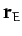and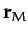be the position vectors of the Earth and Moon, respectively, in a non-rotating reference frame in which the Sun is at rest at the origin. Treating this reference frame as inertial (which is an excellent approximation, given that the mass of the Sun is very much greater than that of the Earth or the Moon--see Chapter 6), the Earth's equation of motion becomes (see Chapter 5)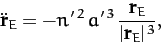(1119)

whereper day and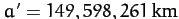are the mean angular velocity and major radius, respectively, of the terrestrial orbit about the Sun. Here,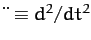. On the other hand, the Moon's equation of motion takes the form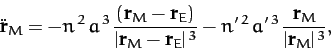(1120)

where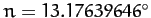per day and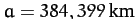are the mean angular velocity and major radius, respectively, of the lunar orbit about the Earth. Note that we have retained the perturbing influence (i.e., acceleration) of the Sun in the lunar equation of motion, (1120), whilst neglecting the perturbing influence of the Moon in the terrestrial equation of motion, (1119), since the former influence is significantly greater (by a factor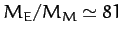, where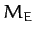is the mass of the Earth, and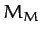the mass of the Moon) than the latter.

Let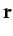(1121)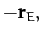(1122)

be the position vectors of the Moon and Sun, respectively, relative to the Earth. It follows, from Equations (1119)-(1122), that in a non-inertial reference frame,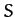(say), in which the Earth is at rest at the origin, but the coordinate axes point in fixed directions, the lunar and solar equations of motion take the form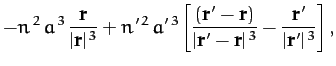(1123)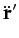(1124)

respectively.

Let us set up a conventional Cartesian coordinate system inwhich is such that the (apparent) orbit of the Sun about the Earth lies in the-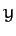plane. This implies that the-plane corresponds to the so-called ecliptic plane. Accordingly, in, the Sun appears to orbit the Earth at the mean angular velocity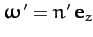(assuming that the-axis points toward the so-called north ecliptic pole), whereas the projection of the Moon onto the ecliptic plane orbits the Earth at the mean angular velocity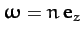.

In the following, for the sake of simplicity, we shall neglect the small eccentricity,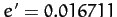, of the Sun's apparent orbit about the Earth (which is actually the eccentricity of the Earth's orbit about the Sun), and approximate the solar orbit as a circle, centered on the Earth. Thus, if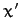,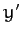,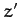are the Cartesian coordinates of the Sun inthen an appropriate solution of the solar equation of motion, (1124), is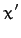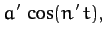(1125)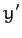(1126)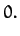(1127)Next: Lunar Equations of Motion Up: Lunar Motion Previous: Historical Background
Richard Fitzpatrick 2011-03-31